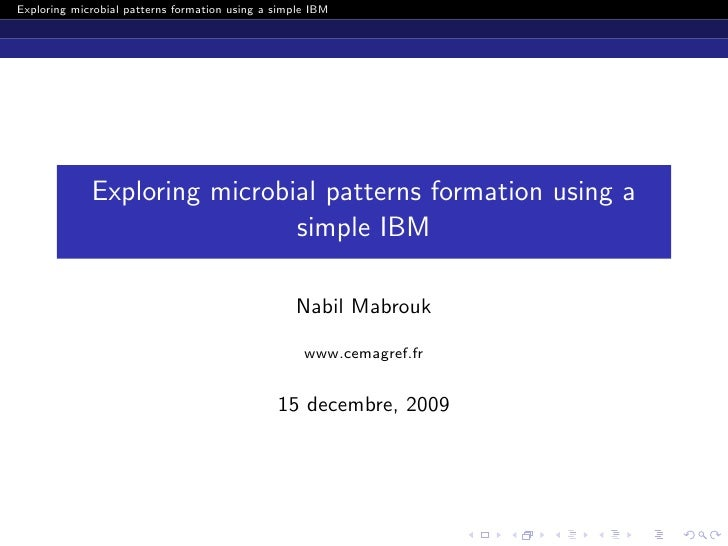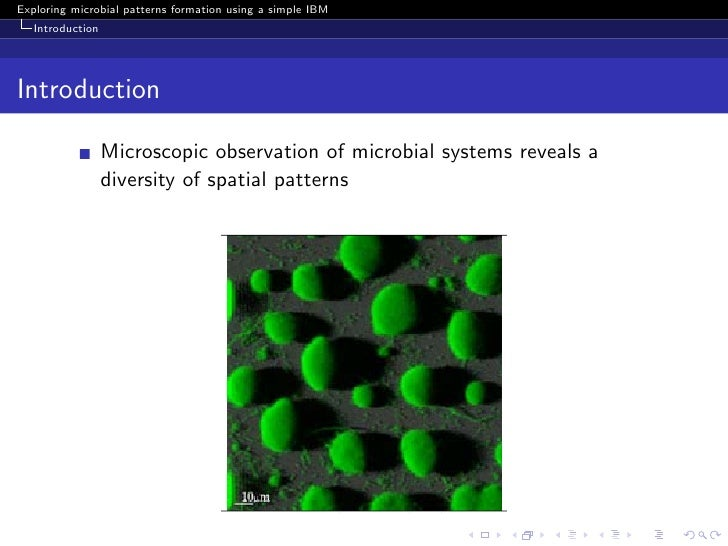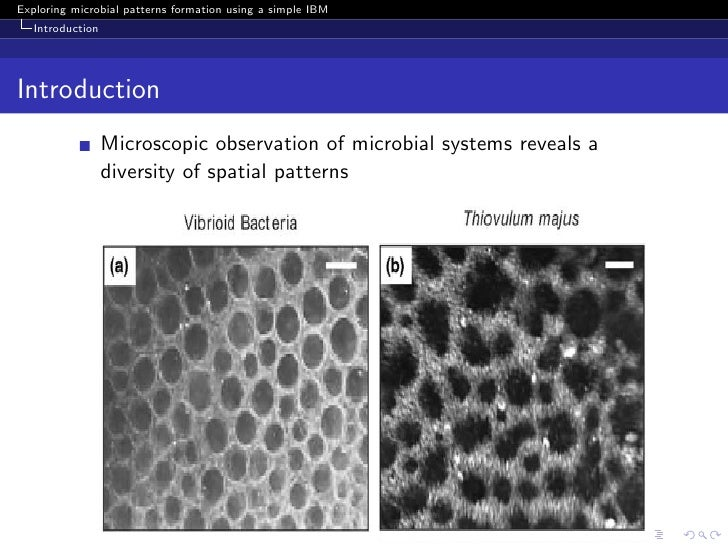Successfully reported this slideshow.Upcoming SlideShare
×

ofUpcoming SlideShare
5 Táticas para Tornar seu Marketing no Instagram mais Efetivo!
Next

Share

# Exploring spatial pattern formation using a simple individual-based model

Bacteria can form intriguing spatial patterns observable at large spatial scales compared to the size of the cell. These patterns are formed through local interactions between the individuals and can impact the dynamic of the population. We illustrate how individual-based models can be used to explore such patterns.

See all

### Related Audiobooks

#### Free with a 30 day trial from Scribd

See all
• Be the first to like this

### Exploring spatial pattern formation using a simple individual-based model

1. 1. Exploring microbial patterns formation using a simple IBM Exploring microbial patterns formation using a simple IBM Nabil Mabrouk www.cemagref.fr 15 decembre, 2009
2. 2. Exploring microbial patterns formation using a simple IBM Introduction Introduction Microscopic observation of microbial systems reveals a diversity of spatial patterns
3. 3. Exploring microbial patterns formation using a simple IBM Introduction Introduction Microscopic observation of microbial systems reveals a diversity of spatial patterns
4. 4. Exploring microbial patterns formation using a simple IBM Introduction Introduction Our aim: investigate how these large-scale patterns emerge
5. 5. Exploring microbial patterns formation using a simple IBM Introduction Introduction Our aim: investigate how these large-scale patterns emerge Our approach: individual-based modeling
6. 6. Exploring microbial patterns formation using a simple IBM Introduction Introduction Our aim: investigate how these large-scale patterns emerge Our approach: individual-based modeling Represent the individuals explicitly
7. 7. Exploring microbial patterns formation using a simple IBM Introduction Introduction Our aim: investigate how these large-scale patterns emerge Our approach: individual-based modeling Represent the individuals explicitly Simulate the pattern formation under diﬀerent conditions
8. 8. Exploring microbial patterns formation using a simple IBM A simple birth-death model Model description Simple is beautiful, and necessary (Deﬀuant et al., 2003)
9. 9. Exploring microbial patterns formation using a simple IBM A simple birth-death model A simple birth-death model Overview: 2D domain with individuals represented as point particles
10. 10. Exploring microbial patterns formation using a simple IBM A simple birth-death model A simple birth-death model Overview: 2D domain with individuals represented as point particles Two processes:
11. 11. Exploring microbial patterns formation using a simple IBM A simple birth-death model A simple birth-death model Overview: 2D domain with individuals represented as point particles Two processes: death with a probability d
12. 12. Exploring microbial patterns formation using a simple IBM A simple birth-death model A simple birth-death model Overview: 2D domain with individuals represented as point particles Two processes: death with a probability d
13. 13. Exploring microbial patterns formation using a simple IBM A simple birth-death model A simple birth-death model Overview: 2D domain with individuals represented as point particles Two processes: death with a probability d birth with a probability b
14. 14. Exploring microbial patterns formation using a simple IBM A simple birth-death model A simple birth-death model Overview: 2D domain with individuals represented as point particles Two processes: death with a probability d birth with a probability b
15. 15. Exploring microbial patterns formation using a simple IBM A simple birth-death model A simple birth-death model Overview: 2D domain with individuals represented as point particles Two processes: death with a probability d birth with a probability b We are interested in the case: wb << L : local birth
16. 16. Exploring microbial patterns formation using a simple IBM A simple birth-death model A simple birth-death model Overview: 2D domain with individuals represented as point particles Two processes: death with a probability d birth with a probability b We are interested in the case: wb << L : local birth b = d = constant
17. 17. Exploring microbial patterns formation using a simple IBM A simple birth-death model A simple birth-death model Overview: 2D domain with individuals represented as point particles Two processes: death with a probability d birth with a probability b We are interested in the case: wb << L : local birth b = d = constant mean-ﬁeld limit (for large N): dN dt = (b − d)N
18. 18. Exploring microbial patterns formation using a simple IBM A simple birth-death model Simulation with wb /L = 0.015 Figure: t = 0
19. 19. Exploring microbial patterns formation using a simple IBM A simple birth-death model Simulation with wb /L = 0.015 Figure: t = 400
20. 20. Exploring microbial patterns formation using a simple IBM A simple birth-death model Simulation with wb /L = 0.1 Figure: t = 400
21. 21. Exploring microbial patterns formation using a simple IBM A simple birth-death model A simple birth-death model Overview: Two processes: death with a probability di , i = 1..N birth with a probability b We are interested in the case: wb << L : local birth birth probability b is constant
22. 22. Exploring microbial patterns formation using a simple IBM A simple birth-death model A simple birth-death model Overview: Two processes: death with a probability di , i = 1..N birth with a probability b We are interested in the case: wb << L : local birth birth probability b is constant death probabilities depend on the neighborhood (the pattern)
23. 23. Exploring microbial patterns formation using a simple IBM A simple birth-death model A simple birth-death model Overview: Two processes: death with a probability di , i = 1..N birth with a probability b We are interested in the case: wb << L : local birth birth probability b is constant death probabilities depend on the neighborhood (the pattern) ||xi −xj || di = d1 + d2 j Kd wb
24. 24. Exploring microbial patterns formation using a simple IBM A simple birth-death model A simple birth-death model Overview: Two processes: death with a probability di , i = 1..N birth with a probability b We are interested in the case: wb << L : local birth birth probability b is constant death probabilities depend on the neighborhood (the pattern) ||xi −xj || di = d1 + d2 j Kd wb wb << wd , b > d1 and d2 > 0
25. 25. Exploring microbial patterns formation using a simple IBM A simple birth-death model Simulation with wb /L = 0.015 and wd >> wb Figure: t = 0
26. 26. Exploring microbial patterns formation using a simple IBM A simple birth-death model Simulation with wb /L = 0.015 and wd >> wb Figure: t = 800
27. 27. Exploring microbial patterns formation using a simple IBM Birth-death model with motility A birth-death model with motility Overview: Three processes: death with a probability di , i = 1..N birth with a probability b motility with a probability mi , i = 1..N
28. 28. Exploring microbial patterns formation using a simple IBM Birth-death model with motility A birth-death model with motility Overview: Three processes: death with a probability di , i = 1..N birth with a probability b motility with a probability mi , i = 1..N
29. 29. Exploring microbial patterns formation using a simple IBM Birth-death model with motility A birth-death model with motility Overview: Three processes: death with a probability di , i = 1..N birth with a probability b motility with a probability mi , i = 1..N
30. 30. Exploring microbial patterns formation using a simple IBM Birth-death model with motility A birth-death model with motility Overview: Three processes: death with a probability di , i = 1..N birth with a probability b motility with a probability mi , i = 1..N We are interested in the case:
31. 31. Exploring microbial patterns formation using a simple IBM Birth-death model with motility A birth-death model with motility Overview: Three processes: death with a probability di , i = 1..N birth with a probability b motility with a probability mi , i = 1..N We are interested in the case: motility probabilities depend on the neighborhood ||xi −xj || mi = m1 −m2 j Kv wv
32. 32. Exploring microbial patterns formation using a simple IBM Birth-death model with motility Parameters 9 parameters: wb , wd , wm , wv b, d1 , d2 , m1 and m2 Additional assumptions: wb (birth) << wd (death) wm (mobility) >> wb (birth) wv (”viscosity’) > wd (death) b >> d1 m1 = 1.0 and d2 , m2 > 0
33. 33. Exploring microbial patterns formation using a simple IBM Birth-death model with motility Simulation results Figure: t = 0
34. 34. Exploring microbial patterns formation using a simple IBM Birth-death model with motility Simulation results Figure: t = 800
35. 35. Exploring microbial patterns formation using a simple IBM Birth-death model with motility Are these patterns realistic? Figure: (Xavier et al., 2009) Fluorescent microscopy of yellow [U+FB02]uorescent protein-labeled bioﬁlm shows cells in spatial patterns with holes, labyrinths, and wormlike shapes.
36. 36. Exploring microbial patterns formation using a simple IBM Birth-death model with motility Are these patterns realistic? Figure: (Xavier et al., 2009) Continuous variation of spatial patterns across the surface of the coverslip is produced by the systematic variation of nutrient concentration. This image is a montage of four contiguous phase-contrast microscopy images.
37. 37. Exploring microbial patterns formation using a simple IBM Conclusion ”A change without pattern is beyond Science” (Zeide, 1991)
38. 38. Exploring microbial patterns formation using a simple IBM Conclusion ”A change without pattern is beyond Science” (Zeide, 1991) Experimental data contains: meaningful pattern and misleading noise
39. 39. Exploring microbial patterns formation using a simple IBM Conclusion ”A change without pattern is beyond Science” (Zeide, 1991) Experimental data contains: meaningful pattern and misleading noise IBM (modeling) can help in extracting patterns and understanding how they form and impact the population
40. 40. Exploring microbial patterns formation using a simple IBM Conclusion ”A change without pattern is beyond Science” (Zeide, 1991) Experimental data contains: meaningful pattern and misleading noise IBM (modeling) can help in extracting patterns and understanding how they form and impact the population Perspectives ...
41. 41. Exploring microbial patterns formation using a simple IBM Conclusion The end!

Total views

1,001

On Slideshare

0

From embeds

0

Number of embeds

7

10

Shares

0